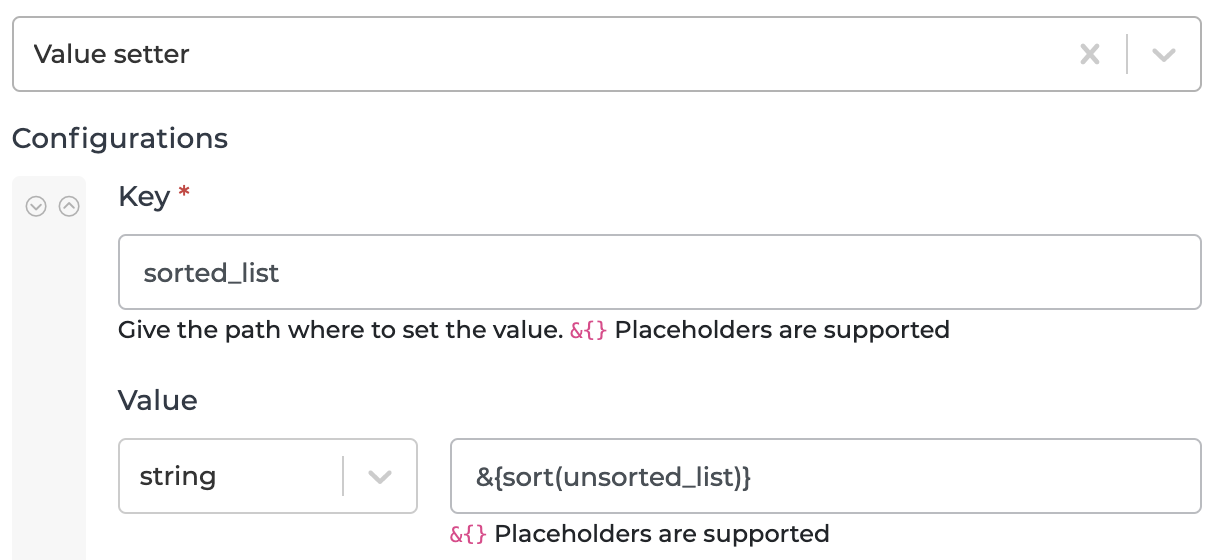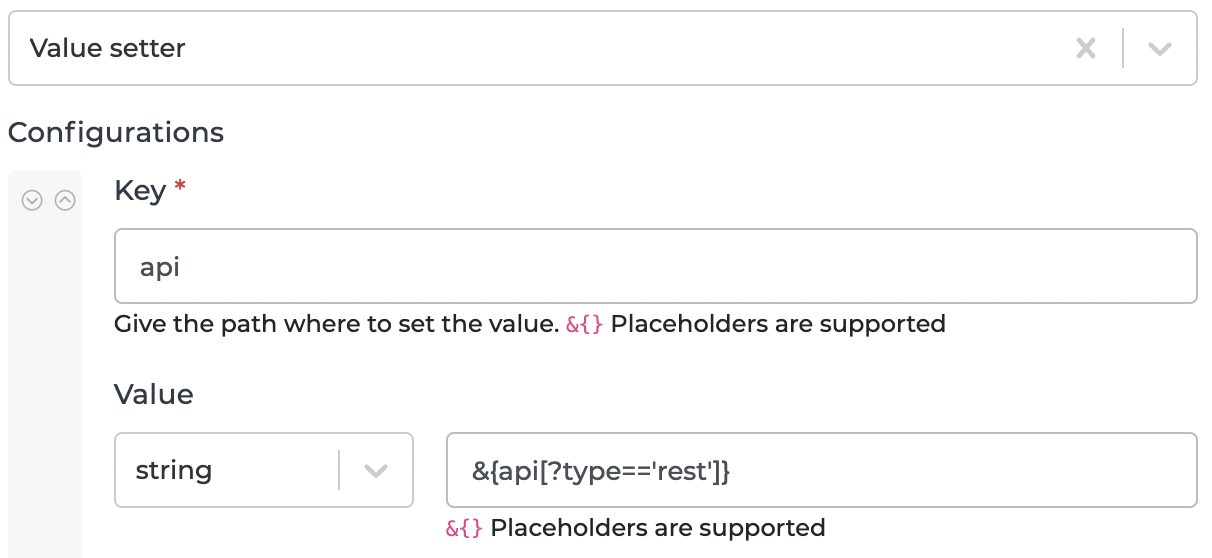# Use JMESPath to simplify your transformations

Did you already know that Alumio supports the JMESPath query language? This enables you to manipulate your data even easier than before, as this allows you to execute code-like methods directly on your data.

Let me provide you with two examples!

Sorting lists

Let’s assume we have the following data:

``````{
"unsorted_list": [10,6,8,12]
}
``````

In order to sort this list, we can use the *sort function (*documentation: JMESPath Specification — JMESPath) to let JMESPath automatically sort this for us. The sample configuration is shown in the image below.The result is as follows:

``````{
"unsorted_list": [10, 6, 8, 12],
"sorted_list": [6, 8, 10, 12]
}
``````

Filtering an array of objects

For this example we will assume the following data:

``````{
"api": [{
"type": "rest",
"name": "Magento 2.4"
},
{
"type": "soap",
"name": "Magento 1.9"
},
{
"type": "rest",
"name": "Shopware 6.4"
}
]
}
``````

Now if we only want to keep the API objects where the type equals rest, we can utilize the filtering capability of JMESPath (documentation can be found here: JMESPath Examples — JMESPath) by applying the following transformation:This brings us the following result:

``````{
"api": [{
"type": "rest",
"name": "Magento 2.4"
},
{
"type": "rest",
"name": "Shopware 6.4"
}
]
}
``````

Comparing Values

JMESPath supports common comparison operators, such as below.

• `==` (equals)
• `!=` (doesn’t equal)
• `<` (is less than)
• `<=` (is less than or equal to)
• `>`, (is greater than)
• `>=` (is greater than or equal to)

Let’s assume we have the following data.

``````{
"val1": "foo",
"val2": "bar",
"val3": "baz"
}
``````

In order to check whether property `val1 ` equals to `foo`, you can create a property called `result`, such as below.

The value of the `result` property will be `true` because the value of the property `val1` does indeed equal to `foo`.

``````{
"val1": "foo",
"val2": "bar",
"val3": "baz",
"result": true
}
``````

In addition to the comparators, JMESPath provides the ability to use logical expressions, such as below.

• `&&` (AND expression),
• `||` (OR expression),
• `!` (NOT expression),
• `(...)` (Paren expressions)

By using the same data as the previous example, we want to check multiple conditions, such as below.

The conditions are `val1` equals to `foo` AND `val2` equals to `bar`, then, the result of previous conditions OR `val3` doesn’t equal to `baz`. We will need the below JMESPath syntax.

``````&{(val1 == 'foo' && val2 == 'bar') || val3 != 'baz'}
``````

The result of the above syntax is `true` because the result of conditions in the parenthesis is `true`. Therefore, it doesn’t matter what is the result of the last condition (because it uses OR expression), the final result will be `true`.

Hopefully this information helped you understanding the basics of JMESPath. More information can be found over at https://jmespath.org/.

Best of luck implementing this within your integrations and feel free to share your own JMESPath tips and tricks below!